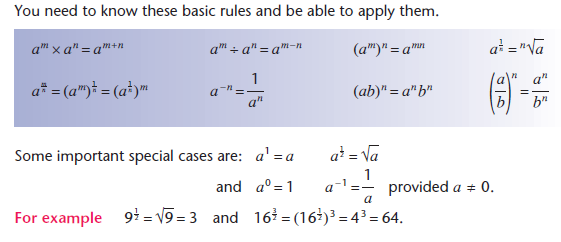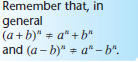## Indices

This section covers Indices revision.An index number is a number which is raised to a power. The power, also known as the index, tells you how many times you have to multiply the number by itself. For example, 25 means that you have to multiply 2 by itself five times = 2×2×2×2×2 = 32.

There are a number of important rules of index numbers:

• ya × yb = ya+b

Examples

24 × 28 = 212

54 × 5-2 = 52

• ya ÷ yb= ya-b

Examples

39 ÷ 34 = 35

72 ÷ 75 = 7-3

• y -b = 1/yb

Examples

2-3 = 1/23 = 1/8

3-1 = 1/3

• ym/n = (n√y)m

Examples

161/2 = √16 = 4

82/3 = (3√8)2 = 4

• (yn)m = ynm

Example

25 + 84

= 25 + (23)4

= 25 + 212

• y0 = 1

Example

50 = 1